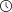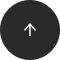- 8 minutes read

# How to Work With the Calendar and Arrow Python Modules

During your journey as a developer or data scientist, you will have to work with dates and times. Fortunately, Python has several convenient libraries that make it easier.

In this article, we’ll quickly review the datetime module, then examine the calendar and Arrow modules in Python.

As a Python-using programmer, developer, data analyst, or data scientist, you’ll probably have to deal with date and time data quite often. Fortunately, Python’s `datetime`, `calendar`, and `arrow` libraries are available to streamline your tasks. Let’s see how they work, and then focus on using the calendar and Arrow libraries in particular.

## How to Use Datetime in Python

We’ve already discussed the datetime module  in this blog, so I will just do a quick review before moving to the calendar and arrow modules.

The `datetime` module offers types like date, time, `datetime`, and `timedelta`. It also lets you retrieve time zone information. For example:

```# import
import datetime

# get current date and time
datetime_now = datetime.datetime.now()
print(datetime_now)
```

Output:

`2021-07-28 15:10:08.773252`

We can also print dates as strings in different formats:

```# Import
from datetime import datetime

# Set a date as a string
date = "11/07/2021 10:25:32"

# Print date as dd/mm/yyyy format
date_obj1 = datetime.strptime(date, "%d/%m/%Y %H:%M:%S")
print("date and time =", date_obj1)

# Print date as mm/dd/yyyy format
date_obj2 = datetime.strptime(date, "%m/%d/%Y %H:%M:%S")
print("date and time =", date_obj2)
```

Output:

```date and time = 11/07/2021 10:25:32
date and time = 11/07/2021 10:25:32
```

The `strftime()` method lets you format time as a readable string. In the following example, we output the month in a readable format:

```# Import
import datetime

# Set a date
date = datetime.datetime(2018, 6, 1)

# Print month in a readable format
print(date.strftime("%B"))
```

Result:

`June`

There’s a handy cheat sheet on `strftime()` here. Feel free to use it. Later, we will see a more efficient way to retrieve this information with the `arrow` module.

The `timedelta()` function lets you perform comparison operations and measure time duration:

```# Import
from datetime import datetime, timedelta

# Using current time
current_time = datetime.now()

# Print current time
print ("Current time: ", str(current_time))

# Calculate future date in three years time
date_aft_3yrs = current_time + timedelta(days = 1095)

# Calculate future date in five days
date_aft_5days = current_time + timedelta(days = 5)

# Print calculated future_dates
print('In three years from now, the date will be:', str(date_aft_3yrs))
print('In five days from now, the date will be:', str(date_aft_5days))
```

We went through naive `datetime` objects in the previous example, i.e. there is no time zone information. However, It’s good practice to work with time-aware objects. We can set the time zone using the `pytz` module, as `datetime` alone is not enough here. If you haven’t installed `pytz` yet, you can do it with `pip`:

`pip install pytz `

Here’s a working example:

```import datetime
import pytz

utc_now = pytz.utc.localize(datetime.datetime.utcnow())
eur_now = utc_now.astimezone(pytz.timezone("Europe/Paris"))

eur_now == utc_now
```

Now we have two time objects with different time zones, but they are equals. We can print them as follows:

`utc_now.isoformat()`

The output of Coordinated Universal Time is:

`'2021-07-28T07:39:51.567436+00:00'`

And here, let’s return the European Time in Paris:

`eur_now.isoformat()`

The output of European Time in Paris is:

`'2021-07-28T09:39:51.567436+02:00'`

For more information about the `datetime` module, you can check the Python datetime documentation.

## How to Use Calendar in Python

Python has an attractive built-in module called `calendar` that lets you conveniently display and work with calendars in Python.

To display the calendar for July 2021, you’d write:

```import calendar

mth_calendar = calendar.TextCalendar(0).formatmonth(2021, 7)
print(mth_calendar)
```

And this is the result:To display the entire 2021 calendar, we can type the following:

```import calendar

yr_calendar = calendar.TextCalendar(0).formatyear(2021)
print(yr_calendar)
```We can also change the first weekday (Monday / Sunday) by modifying the `firstweekday` parameter. We do this by entering an integer between `0` and `6`, where Monday = `0` and Sunday = `6`.

```import calendar

# Set Thursday as first day of the month
mth_calendar = calendar.TextCalendar(firstweekday=3).formatmonth(2021, 7)
print(mth_calendar)
```

Also, by changing `TextCalendar` to `HTMLCalendar`, we can display the calendar in HTML form. It will return the calendar with its corresponding HTML tags to display on a webpage. The syntax is as follows:

```import calendar

mth_calendar = calendar.HTMLCalendar(firstweekday=0).formatmonth(2021, 7)
print(mth_calendar)
```

Next, let’s see how `calendar.monthrange(year, month)` returns the first day of the month and the number of days in that particular month. For example:

`calendar.monthrange(2021, 8)`

Output:

`(6, 31)`

This means that the first day of August 2021 is a Sunday and that there are 31 days in the month.

We can also use the `isleap()` method to check if a year is a leap year. It will return a Boolean value of True if the value is a leap year and False if it is not.  Here is an example:

```import calendar

print(calendar.isleap(2021))
```

And the result:

`False`

Next, `calendar.weekday(year, month, day)` will return the weekday of a given date. For example, what was the weekday on 14 July 1789, the date chosen to commemorate the French National Day?

```import calendar

calendar.weekday(1789, 7, 14)
```

Output:

`1`

It was a Tuesday.

If you want to explore the calendar module in greater detail, you will find the official documentation here.

## How to Use Arrow in Python

Arrow is another great Python library, named after the Arrow of Time. This is the concept of time’s one-way direction or "asymmetry". The Arrow of Time refers to how we always see things progressing in a particular (one-way) direction. It refers to the inevitable "flow of time" into the future.

Unlike `datetime` and `calendar`, which are built-in modules, `arrow` needs to be installed. You can do this with `pip`:

`pip install arrow `

We can use `arrow` to get the Coordinated Universal Time:

```import arrow

utc = arrow.utcnow()
print(utc)
```

The output is:

`2021-07-28T14:04:48.910069+00:00`

Also, we can use arrow to get the local time:

```import arrow

now = arrow.now()
print(now)
```

Result:

`2021-07-28T22:06:14.795852+08:00`

If we want to parse a string into a date format, we need to use `get()`:

```# Import
import arrow

# Date in string format
date_str ='2021-07-20 15:20:35'

# Parse string into date
date_f = arrow.get(date_str, 'YYYY-MM-DD HH:mm:ss')

# Print the date
print(date_f)
```

Output:

`2021-07-20T15:20:35+00:00`

Note that Arrow uses simpler, shorter syntax for parsing the date than `datetime`'s `strptime()`.

It is also possible to retrieve a date from a string like the sentence below:

```# Import
import arrow

# Retrieve date from string
get_dt_f_str = arrow.get('Olympic games started on 23 July 2021', 'D MMMM YYYY')

# Print date from string
print(get_dt_f_str)
```

Result:

`2021-07-23T00:00:00+00:00`

Next, we can use `format()` to format a date as a string:

```# Import
import arrow

# Get local time
now = arrow.now()

# Print the year
year = now.format('YYYY')
print("Year: {0}".format(year))

# Print the date as year, month, day
date = now.format('YYYY-MM-DD')
print("Date: {0}".format(date))

# Print the date and the time
date_time = now.format('YYYY-MM-DD HH:mm:ss')
print("Date and time: {0}".format(date_time))

# Print the date, time and time zone
date_time_zone = now.format('YYYY-MM-DD HH:mm:ss ZZ')
print("Date and time and zone: {0}".format(date_time_zone))
```

Output:

```Year: 2021
Date: 2021-07-28
Date and time: 2021-07-28 22:12:02
Date and time and zone: 2021-07-28 22:12:02 +08:00
```

We can also retrieve a weekday:

```# Import
import arrow

# Get the date
bastille_day = arrow.get('1789-07-14')

# Print the weekday and format it in a readable way
print(bastille_day.weekday())
print(bastille_day.format('dddd'))
```

Output:

```1
Tuesday
```

We can also get the time in another part of the world via conversion. Here is an example:

```# Import
import arrow

# Get local time
utc = arrow.utcnow()

# Get time in different parts of the world
print("Time in US/Pacific:", utc.to('US/Pacific').format('HH:mm:ss'))
print("Time in Europe/Paris:", utc.to('Europe/Paris').format('HH:mm:ss'))
print("Time in Asia/Tokyo:",utc.to('Asia/Tokyo').format('HH:mm:ss'))
```

Output:

```Time in US/Pacific: 07:18:37
Time in Europe/Paris: 16:18:37
Time in Asia/Tokyo: 23:18:37
```

Next, let’s shift time:

```# Import
import arrow

# Get current time
now = arrow.now()

# Shift time 3 hours into the future
print("In 3 hours, it will be:", now.shift(hours=3).time())

# Shift time 3 days into the future
print("In 3 days, the date will be:", now.shift(days=3).date())

# Shift time 5 years into the past
print("5 years ago, the date was:", now.shift(years=-5).date())
```

Output:

```In 3 hours, it will be: 01:23:20.609249
In 3 days, the date will be: 2021-07-31
5 years ago, the date was: 2016-07-28
```

You can mix different arguments together, e.g., `now.shift(days=3, hours=6)`.

Also, we might be interested in formatting time for humans so that it’s more readable than the long strings we’ve been getting.

The `humanize()` method is a convenient and powerful way to achieve a human-readable output. Let’s write an example:

```# Import
import arrow

# Get current time
now = arrow.now()

moment_ago = now.shift(minutes=-15).humanize()
print(moment_ago)

future_moment = now.shift(hours=5).humanize()
print(future_moment)
```

Output:

```15 minutes ago
in 5 hours
```

On the other hand, we can use `dehumanize()` to make a date more machine-friendly:

```# Import
import arrow

# Get current time
current_time = arrow.utcnow()

# Set time two days ago
two_days_ago = current_time.dehumanize("2 days ago")
print(two_days_ago)
```

Result:

`2021-07-26T14:31:33.975369+00:00`

The `arrow` module offers a more readable output than `datetime` and `calendar`.

Compared to `datetime`, arrow makes creating, manipulating, formatting, and converting dates, time, timestamps, time zones, etc. more straightforward. It requires less code and less dependence on other imports or libraries. Overall, using the arrow module is more efficient than Python’s built-in modules.

Finally, it is always a good idea to read the official documentation when we learn something new.

## Practice Working with Python’s Date and Time Modules

We’ve reviewed the `datetime` module and covered the `calendar` and arrow modules in Python. That is quite a bit of ground, and I hope it helped you understand how to manipulate date and time data with Python.

Try running these code snippets and playing with them to solidify your knowledge.

If you want to get some hands-on experience working with Python’s data science modules, I recommend the LearnSQL.com Python for Data Science mini-track. Its five courses and 300+ exercises will give you plenty of practice! Or check out our Data Processing with Python mini-track, which comprehensively explains how to process various kinds of data in different file formats. Happy learning!